While some posts in our Minitab blog focus on understanding t-tests and t-distributions this post will focus more simply on how to hand-calculate the t-value for a one-sample t-test (and how to replicate the p-value that Minitab gives us).

The formulas used in this post are available within Minitab Statistical Software by choosing the following menu path: Help > Methods and Formulas > Basic Statistics > 1-sample t.

The null and three alternative hypotheses for a one-sample t-test are shown below: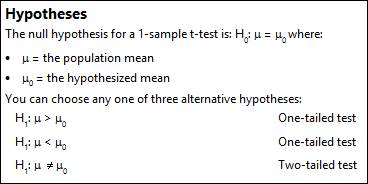The default alternative hypothesis is the last one listed: The true population mean is not equal to the mean of the sample, and this is the option used in this example.To understand the calculations, we’ll use a sample data set available within Minitab.  The name of the dataset is Bears.MTW, because the calculation is not a huge bear to wrestle (plus who can resist a dataset with that name?).

For this example, we will use column C2, titled Age, in the Bears.MTW data set, and we will test the hypothesis that the average age of bears is 40. First, we’ll use Stat > Basic Statistics > 1-sample t to test the hypothesis: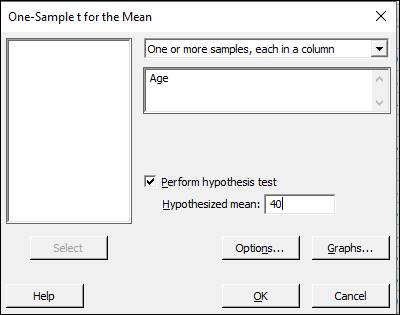After clicking OK above we see the following results in the session window: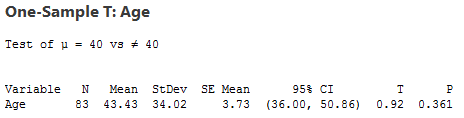With a high p-value of 0.361, we don’t have enough evidence to conclude that the average age of bears is significantly different from 40.

Now we’ll see how to calculate the T value above by hand.

The formula for the T value (0.92) shown above is calculated using the following formula in Minitab: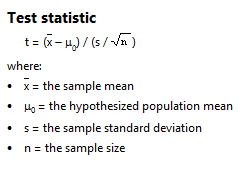The output from the 1-sample t test above gives us all the information we need to plug the values into our formula:

Sample mean: 43.43

Sample standard deviation: 34.02

Sample size: 83

We also know that our target or hypothesized value for the mean is 40.

Using the numbers above to calculate the t-statistic we see:

t = (43.43-40)/34.02/√83) = 0.918542
(which rounds to 0.92, as shown in Minitab’s 1-sample t-test output)

Now, we could dust off a statistics textbook and use it to compare our calculated t of 0.918542 to the corresponding critical value in a t-table, but that seems like a pretty big bear to wrestle when we can easily get the p-value from Minitab instead.  To do that, I’ve used Graph > Probability Distribution Plot > View Probability: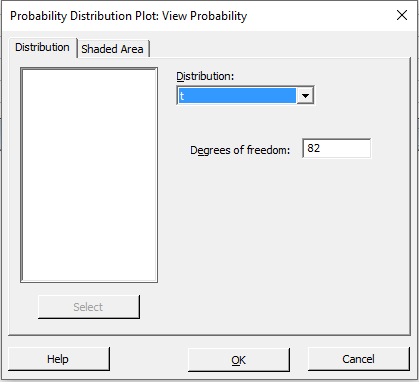In the dialog above, we’re using the t distribution with 82 degrees of freedom (we had an N = 83, so the degrees of freedom for a 1-sample t-test is N-1).  Next, I’ve selected the Shaded Area tab: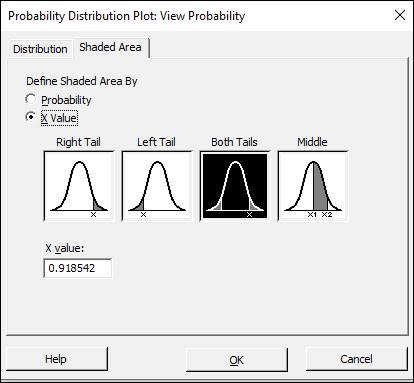In the dialog box above, we’re defining the shaded area by the X value (the calculated t-statistic), and I’ve typed in the t-value we calculated in the X value field. This was a 2-tailed test, so I’ve selected Both Tails in the dialog above.

After clicking OK in the window above, we see: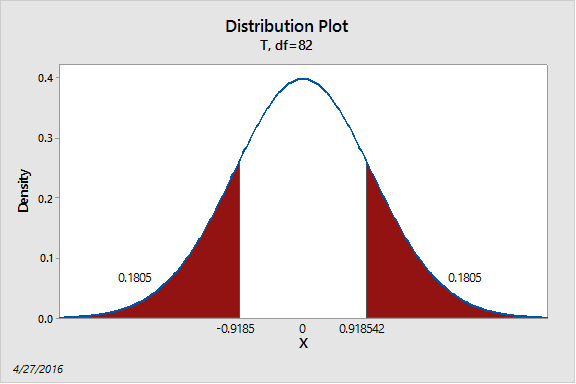We add together the probabilities from both tails, 0.1805 + 0.1805 and that equals 0.361 – the same p-value that Minitab gave us for the 1-sample t test.

That wasn’t so bad—not a difficult bear to wrestle at all!